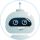# Magic Squares Theory

• Subject
• Category
• Pages 2
• Words 376
• Views 528
• Pages 2
• Words 376
• Views 528

Get original paper in 3 hours and nail the task

124 experts online

### Introduction

Magic square is known for mathematical recreation giving entertainment and an interesting outlet for creating mathematical knowledge.

An nth-order magic square is a square array of n2 distinct integers in which the sum of the n numbers in each row, column, and diagonal is the same.Magic squares history started around 2200 B.C. from China to India, then to the Arab countries.

The first known mathematical use of magic squares in India was by Thakkura Pheru in his work Ganitasara (ca. 1315 A.D.).

Pheru gave a method for constructing odd magic squares, that is to say, squares, where, n is an odd integer. We begin by putting the number 1 in the bottom cell of the central column (as illustrated below). Where by to arrive at the next cell above it, add n + 1, getting n + 2. And the next cell up n + 2, add n + 1 again, getting 2n + 3.

Continue to add in this way to arrive at the cell values in the central column results in an arithmetic progression with a common difference of n + 1. Continue adding n + 1 until arriving at the central column’s top cell, of the value n2.ÂThe first steps in Pheru’s method for constructing odd-order magic squaresOther cells in the square are derived by starting from the numbers in the central column. The diagram above illustrates Pheru’s method.

When making a 9-by-9 magic square, hence n = 9. Take any number in the central column, say, 1. Add n to 1, obtaining9 + 1 = 10. Then move as a knight in chess would, starting at 1 and moving one cell to the left, then two cells up.

In this cell, place the 10. From this cell, repeat the same process. Add 10 + 9 to get 19, complete the knight move, and put 19 in the resulting cell. Further this process by arriving at the cell with a number of 37.

Add 9 and completing the next process puts 46 outside of the original 9-by-9 square. To solve this situation, assume you have 9-by-9 squares on each side and corner of the original 9-by-9 square. You will observe that the cell where 46 is present is in the outside square on top the original square and off to the left-hand corner. Simplifying futher move 46 to the corresponding cell in the original 9-by-9 square.

### Reference

1. http://illuminations.nctm.org/Lessons.aspx ( Visited 24 Novemeber, 2007)

This essay was written by a fellow student. You may use it as a guide or sample for writing your own paper, but remember to cite it correctly. Don’t submit it as your own as it will be considered plagiarism.

## Need a custom essay sample written specially to meet your requirements?

Choose skilled expert on your subject and get original paper with free plagiarism report

Without paying upfront

### Related Topics